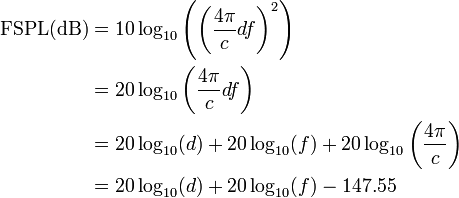## FM Stereo vs station coverage

There are a few FM stations around here that intentionally broadcast in mono.  One is an FM talker, which from a technical standpoint makes a certain amount of sense since any particular human voice is a single point sound generator.

The other FM station broadcasting in mono, WKZE,  has a music format with a very eclectic play list.  It is a full Class A located in north western Connecticut.  The idea with this station is to garner a larger and more reliable coverage area.

It comes down to a simple physics discussion about free space loss.  The basic equation for free space power loss is:where:

•is the signal wavelength (in metres),
•is the signal frequency (in hertz),
•is the distance from the transmitter (in metres),
•is the speed of light in a vacuum, 2.99792458 × 108 metres per second.

That formula works for a single frequency, say the carrier frequency, for example. As the signal gets spread out by modulation, the power density on any given frequency is reduced as the energy is divided between many other frequencies.

First, free space loss takes into account the spreading out of electromagnetic energy in free space is determined by the inverse square law, i.e.where:

•is the power per unit area or power spatial density (in watts per metre-squared) at distance,
•is the total power transmitted (in watts).

Second, with Frequency Modulation (FM), the power spectral density is a function of the differences in the highest and lowest frequency:Therefore, the narrower the bandwidth of a signal, the higher the density of the received signal will be in relation to the transmitted power.  An unmodulated FM signal will have a better, more reliable coverage area than a modulated one.  Of course, we need to modulate the signal, otherwise there is no point in having the transmitter on.

A baseband or composite FM signal has several components: